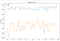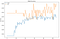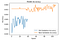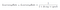Can neural networks be used for binary classification in the case of unbalanced datasets?

There are a few ways to address unbalanced datasets: from built-in class_weight in a logistic regression and sklearn estimators to manual oversampling, and SMOTE. We will look at whether neural networks can serve as a reliable out-of-the-box solution and what parameters can be tweaked to achieve a better performance.

Code is available on GitHub.

We’ll use the Framingham Heart Study data set from Kaggle for this exercise. It presents a binary classification problem in which we need to predict a value of the variable “TenYearCHD” (zero or one) that shows whether a patient will develop a heart disease. The majority (~85%) of the patients don’t have a condition, so it’s exactly the kind of a situation we’re interested in exploring.

The dataset requires some cleansing that is out of the scope of this article and is discussed extensively here and here. That said, I’ll just put the required code below:

import pandas as pd
import numpy as np
import matplotlib.pyplot as plt
import scipy.stats as st
import seaborn as sns
import pandas_profiling
%matplotlib inline
from google.colab import files
import io
# Exploring cigsPerDay
df['cigsPerDay'].value_counts(normalize = True).plot(kind="bar")
df['cigsPerDay'][df['currentSmoker']==0].isna().sum()
# creating a boolean array of smokers
smoke = (df['currentSmoker']==1)
# applying mean to NaNs in cigsPerDay but using a set of smokers only
df.loc[smoke,'cigsPerDay'] = df.loc[smoke,'cigsPerDay'].fillna(df.loc[smoke,'cigsPerDay'].mean())
df['cigsPerDay'][df['currentSmoker']==1].mean()
# Filling out missing values
df['BPMeds'].fillna(0, inplace = True)
df['glucose'].fillna(df.glucose.mean(), inplace = True)
df['totChol'].fillna(df.totChol.mean(), inplace = True)
df['education'].fillna(1, inplace = True)
df['BMI'].fillna(df.BMI.mean(), inplace = True)
df['heartRate'].fillna(df.heartRate.mean(), inplace = True)
df.isna().sum()

The next step is to create train and test splits:

features = df.iloc[:,:-1]
result = df.iloc[:,-1]
# Splitting the dataset into the Training set and Test set
from sklearn.model_selection import train_test_split
X_train, X_test, y_train, y_test = train_test_split(features, result, test_size = 0.2, random_state = 0)
# Scaling the whole dataset for possible K-fold validation:
X_scaled = sc.fit_transform(features)

Moving to the network itself:

from keras.models import Sequential
from keras.layers import Dense
from keras.callbacks import EarlyStopping

We will start with a basic Sequential model with three layers:

classifier = Sequential()classifier.add(Dense(units = 8, kernel_initializer = 'uniform', activation = 'relu', input_dim= 15))
# Adding the second hidden layer
classifier.add(Dense(units = 8, kernel_initializer = 'uniform', activation = 'relu'))
# Adding the output layer
classifier.add(Dense(units = 1, kernel_initializer = 'uniform', activation = 'sigmoid'))
# Compiling the ANN
# classifier.compile(optimizer = 'adam', loss = 'binary_crossentropy', metrics = ['categorical_accuracy'])
classifier.compile(optimizer = 'adam', loss = 'binary_crossentropy', metrics = ['sparse_categorical_accuracy', 'categorical_accuracy','binary_accuracy', 'accuracy'])

The input data is vectors, and the labels are scalars. I’m choosing a fully connected (Dense) layer with a relu activation. The parameter units is the number of hidden units in this layer. In order to start with something, we are going to use 8. Input_dim provides information about the shape of your input. 15 is the number of features. You can easily check it for yourself:

features.shape
#or
X_train.shape

Keras also allows you to pass input_shape() instead, and it should contain a tuple describing your data. In our scenario, I could have also used input_shape((15,)).

The second layer is similar to the first one. The final layer uses a sigmoid function because I want to get probability scores between 0 and 1 (that a given patient will have a heart condition). Later on, you will be able to round the probabilities to zeroes or ones depending on the desired threshold.

The next step is to compile the network, i.e. configure the future learning process. As a result, a Python object that builds an NN will be created. Keras supports various kinds of optimizers, and they can be further adjusted. We will start with Adam in our case. A loss function will be binary_crossentropy that is optimized for binary classification tasks. Finally, you can track various metrics by passing a list in metrics.

Then we will fit the model, make predictions, and check how accurate they are:

# Fitting the ANN to the training set
history1 = classifier.fit(X_train, y_train, validation_split=0.2, batch_size = 10, epochs = 300, verbose = 0)

In the fit portion, I’ve added validation_split, which takes care of the validation process. The object history1 (returned by classifier.fit) contains a dictionary with the values of metrics (one for training and one for validation) that were chosen during the compile portion. It can be accessed like any other dictionary:

history1.history.keys()

It’s often more convenient to explore the results when they’re plotted:

plt.plot(history1.history['acc'])
plt.plot(history1.history['val_acc'])
plt.title('Model Accuracy')
plt.ylabel('Accuracy')
plt.xlabel('Epoch')
plt.legend(['train', 'val'], loc='upper left')
plt.show()
plt.plot(history1.history['loss'])
plt.plot(history1.history['val_loss'])
plt.title('Model Loss')
plt.ylabel('Loss')
plt.xlabel('Epoch')
plt.legend(['train', 'val'], loc='upper left')
plt.show()
plt.plot(history1.history['binary_accuracy'])
plt.plot(history1.history['val_binary_accuracy'])
plt.title('Binary Accuracy')
plt.ylabel('Binary Accuracy')
plt.xlabel('Epoch')
plt.legend(['train', 'val'], loc='upper left')
plt.show()

Here’s a fairly interesting observation: our very first — and basic — model already overfits! We might have overoptimized some of the parameters. As a result, after the 60-70th epoch, the accuracy on the validation dataset starts decreasing, while the loss goes up. Maybe we don’t need so many epochs and should stop the fitting process a little bit earlier? Let’s find out:

es = EarlyStopping(monitor='val_acc', mode='auto', verbose=0, patience=50)
history2 = classifier.fit(X_train, y_train, validation_split=0.2, batch_size = 10, epochs = 150, verbose = 0, callbacks=[es])
plt.figure(figsize=(12,8))
plt.plot(history2.history['acc'])
plt.plot(history2.history['val_acc'])
plt.title('Model Accuracy')
plt.ylabel('Accuracy')
plt.xlabel('Epoch')
plt.legend(['train', 'val'], loc='upper left')
plt.show()
plt.figure(figsize=(12,8))
plt.plot(history2.history['loss'])
plt.plot(history2.history['val_loss'])
plt.title('Model Loss')
plt.ylabel('Loss')
plt.xlabel('Epoch')
plt.legend(['train', 'val'], loc='upper left')
plt.show()
plt.figure(figsize=(12,8))
plt.plot(history2.history['binary_accuracy'])
plt.plot(history2.history['val_binary_accuracy'])
plt.title('Model Accuracy')
plt.ylabel('Accuracy')
plt.xlabel('Epoch')
plt.legend(['train', 'val'], loc='upper left')
plt.show()

I’m introducing an EarlyStopping callback that interrupts training once a target metric stops improving for a certain number of epochs that is controlled by patience. After that, we can print out updated charts.Validation set can still do better

While we saved computer resources with early stopping, the 85% threshold hasn’t been achieved.

Another well-known method to deal with overfitting is L1/L2 regularization. Let’s explore!

from keras import regularizersclassifier_l2 = Sequential()classifier_l2.add(Dense(units = 8, kernel_initializer = 'uniform', kernel_regularizer = regularizers.l2(0.001), activation = 'relu', input_dim= 15))
# Adding the second hidden layer
classifier_l2.add(Dense(units = 8, kernel_initializer = 'uniform', kernel_regularizer = regularizers.l2(0.001), activation = 'relu'))
# Adding the output layer
classifier_l2.add(Dense(units = 1, kernel_initializer = 'uniform', activation = 'sigmoid'))
# Compiling the ANN

classifier_l2.compile(optimizer = 'adam', loss = 'binary_crossentropy', metrics = ['sparse_categorical_accuracy', 'categorical_accuracy','binary_accuracy', 'accuracy'])
es = EarlyStopping(monitor='val_acc', mode='auto', verbose=0, patience=50)
history_l2 = classifier_l2.fit(X_train, y_train, validation_split=0.2, batch_size = 10, epochs = 200, verbose = 0, callbacks=[es])
# plt.figure(figsize=(12,8))
plt.plot(history_l2.history['acc'])
plt.plot(history_l2.history['val_acc'])
plt.title('Model Accuracy')
plt.ylabel('Accuracy')
plt.xlabel('Epoch')
plt.legend(['train', 'val'], loc='upper left')
plt.show()
plt.figure(figsize=(12,8))
plt.plot(history_l2.history['loss'])
plt.plot(history_l2.history['val_loss'])
plt.title('Model Loss')
plt.ylabel('Loss')
plt.xlabel('Epoch')
plt.legend(['train', 'val'], loc='upper left')
plt.show()
plt.figure(figsize=(12,8))
plt.plot(history_l2.history['binary_accuracy'])
plt.plot(history_l2.history['val_binary_accuracy'])
plt.title('Model Binary Accuracy')
plt.ylabel('Binary Accuracy')
plt.xlabel('Epoch')
plt.legend(['train', 'val'], loc='upper left')
plt.show()

The results are drastically different:Accuracy on the validation set is improving

If you compare the old and the new chart:

# Comparing old and new chartsplt.plot(history2.history['val_acc'])
plt.plot(history_l2.history['val_acc'])
plt.title('Model Accuracy')
plt.ylabel('Accuracy')
plt.xlabel('Epoch')
plt.legend(['Old Validation Accuracy', 'New Validation Accuracy'], loc='lower right')
plt.show()
plt.figure(figsize=(12,8))
plt.plot(history2.history['val_loss'])
plt.plot(history_l2.history['val_loss'])
plt.title('Model Loss')
plt.ylabel('Loss')
plt.xlabel('Epoch')
plt.legend(['Old Validation Loss', 'New Validation Loss'], loc='upper right')
plt.show()
plt.figure(figsize=(12,8))
plt.plot(history2.history['val_binary_accuracy'])
plt.plot(history_l2.history['val_binary_accuracy'])
plt.title('Model Binary Accuracy')
plt.ylabel('Binary Accuracy')
plt.xlabel('Epoch')
plt.legend(['Old Binary Accuracy', 'New Binary Accuracy'], loc='upper left')
plt.show()The new model was trained on fewer epochs, that’s why the orange chart is longer

So, we have already achieved a better accuracy rate than the original model and have also surpassed the required threshold of 85%. You can predict the test set now:

# Making predictions
y_pred_l2 = classifier_l2.predict(X_test)
threshold = 0.4
y_pred_l2 = (y_pred_l2 < threshold).astype(np.int)

You can speculate about the best threshold. Most often the best threshold depends on the nature of your problem. In our case, it’s probably better to falsely diagnose a disease and later find out that it’s a mistake than overlook the problem whatsoever. That said, the number of false negatives should ideally be low. It can be controlled by looking at recall_score (TP/(TP+FN)):

from sklearn.metrics import recall_scorerecall_score(y_test, y_pred_l2)

The existing model returns 96% as its recall score.

Overall, it seems that we were able to resolve the overfitting issue. If it wasn’t enough, we would combine the L2 regularization with dropouts:

classifier_l2_drop = Sequential()from keras.layers import Dropout# rebuilding this time doing dropout for every layer
classifier_l2_drop.add(Dense(units = 8, kernel_initializer = 'uniform', kernel_regularizer = regularizers.l2(0.001), activation = 'relu', input_dim= 15))
classifier_l2_drop.add(Dropout(p=0.1)) # meaning 10% will be dropped duting the learning stage
classifier_l2_drop.add(Dense(units = 8, kernel_initializer = 'uniform', kernel_regularizer = regularizers.l2(0.001), activation = 'relu'))
classifier_l2_drop.add(Dense(units = 1, kernel_initializer = 'uniform', activation = 'sigmoid'))

classifier_l2_drop.compile(optimizer = 'adam', loss = 'binary_crossentropy', metrics = ['sparse_categorical_accuracy', 'categorical_accuracy','binary_accuracy', 'accuracy'])
# Fitting the ANN to the Training set
classifier_l2_drop.fit(X_train, y_train, batch_size = 10, epochs = 300, verbose = 0)
# No early stopping
history_l2_drop = classifier_l2_drop.fit(X_train, y_train, validation_split=0.2, batch_size = 10, epochs = 200, verbose = 0)
# plt.figure(figsize=(12,8))
plt.plot(history_l2_drop.history['acc'])
plt.plot(history_l2_drop.history['val_acc'])
plt.title('Model Accuracy')
plt.ylabel('Accuracy')
plt.xlabel('Epoch')
plt.legend(['train', 'val'], loc='upper left')
plt.show()
# plt.figure(figsize=(12,8))
plt.plot(history_l2_drop.history['loss'])
plt.plot(history_l2_drop.history['val_loss'])
plt.title('Model Loss')
plt.ylabel('Loss')
plt.xlabel('Epoch')
plt.legend(['train', 'val'], loc='upper left')
plt.show()
# plt.figure(figsize=(12,8))
plt.plot(history_l2_drop.history['binary_accuracy'])
plt.plot(history_l2_drop.history['val_binary_accuracy'])
plt.title('Model Binary Accuracy')
plt.ylabel('Binary Accuracy')
plt.xlabel('Epoch')
plt.legend(['train', 'val'], loc='upper left')
plt.show()
# Comparing the existing and the previous modelsplt.plot(history2.history['val_acc'])
plt.plot(history_l2_drop.history['val_acc'])
plt.title('Models'' Accuracy')
plt.ylabel('Accuracy')
plt.xlabel('Epoch')
plt.legend(['Old Validation Accuracy', 'New Validation Accuracy with Dropout'], loc='lower right')
plt.show()
# plt.figure(figsize=(12,8))
plt.plot(history2.history['val_loss'])
plt.plot(history_l2_drop.history['val_loss'])
plt.title('Models'' Loss')
plt.ylabel('Loss')
plt.xlabel('Epoch')
plt.legend(['Old Validation Loss', 'New Validation Loss with Dropout'], loc='upper right')
plt.show()
# plt.figure(figsize=(12,8))
plt.plot(history2.history['val_binary_accuracy'])
plt.plot(history_l2_drop.history['val_binary_accuracy'])
plt.title('Models'' Binary Accuracy')
plt.ylabel('Binary Accuracy')
plt.xlabel('Epoch')
plt.legend(['Old Binary Accuracy', 'New Binary Accuracy with Dropout'], loc='lower right')
plt.show()

After the overfitting is taken care of, we can work on improving the performance further. Let’s try tweaking the learning rate schedule. If you’ve ever used the SGD class, you might have seen such parameters as decay and lr. These are our optimization targets:How Learning Rate can be controlled

This is how it can be implemented:

classifier_l2_lr = Sequential()# rebuilding this time with the learning rate schedule and L2 regularization
classifier_l2_lr.add(Dense(units = 8, kernel_initializer = 'uniform', kernel_regularizer = regularizers.l2(0.001), activation = 'relu', input_dim= 15))
classifier_l2_lr.add(Dropout(p=0.1)) # meaning 10% will be dropped duting the learning stage
classifier_l2_lr.add(Dense(units = 8, kernel_initializer = 'uniform', kernel_regularizer = regularizers.l2(0.001), activation = 'relu'))
classifier_l2_lr.add(Dense(units = 1, kernel_initializer = 'uniform', activation = 'sigmoid'))
epochs = 200
learning_rate = 0.1
decay_rate = learning_rate / epochs
momentum = 0.85
sgd_lr = SGD(lr=learning_rate, momentum=momentum, decay=decay_rate, nesterov=False)
classifier_l2_lr.compile(optimizer = sgd_lr, loss = 'binary_crossentropy', metrics = ['sparse_categorical_accuracy', 'categorical_accuracy','binary_accuracy', 'accuracy'])# Fitting the ANN to the Training set
history_l2_lr = classifier_l2_lr.fit(X_train, y_train, validation_split = 0.2, batch_size = 10, epochs = epochs, verbose = 0)

As you can see, we initialized the starting number of epochs, the learning rate, the decay rate, and the momentum manually and passed them into sgd_lr to use as an optimizer in the compile stage. It’s generally recommended to start with a larger learning rate and momentum than you would use in a normal scenario.

So far, everything we did was geared toward improving the model itself: first, handling overfitting, second, increasing its accuracy. We haven’t tried any methods that are specific to imbalanced datasets. Let’s see whether anything can help us. One of the simplest things to try will be class_weight. Think of it as oversampling.

from sklearn.utils.class_weight import compute_sample_weightclass_wt = compute_sample_weight(class_weight = 'balanced', y = y_train)classifier_l2_wt = Sequential()
classifier_l2_wt.add(Dense(units = 8, kernel_initializer = 'uniform', kernel_regularizer = regularizers.l2(0.001), activation = 'relu', input_dim= 15))
# Adding the second hidden layer
classifier_l2_wt.add(Dense(units = 8, kernel_initializer = 'uniform', kernel_regularizer = regularizers.l2(0.001), activation = 'relu'))
# Adding the output layer
classifier_l2_wt.add(Dense(units = 1, kernel_initializer = 'uniform', activation = 'sigmoid'))
# Compiling the ANN
# classifier.compile(optimizer = 'adam', loss = 'binary_crossentropy', metrics = ['categorical_accuracy'])
classifier_l2_wt.compile(optimizer = 'adam', loss = 'binary_crossentropy', metrics = ['sparse_categorical_accuracy', 'categorical_accuracy','binary_accuracy', 'accuracy'])
# Making predictions
y_pred_l2_lr = classifier_l2_lr.predict(X_test)
threshold = 0.4
y_pred_l2_lr = (y_pred_l2_lr < threshold).astype(np.int)
cm_l2_lr = confusion_matrix(y_test, y_pred_l2_lr)
cm_l2_lr

In summary, you can combine various approaches together — let’s say, dropout and learning schedule, or early stopping, L2 regularization, and class_weight.

In addition, you might start with a smaller network (units = 4 in the first and second layers), change the optimizer from Adam to rmsprop, or, if you have enough computing power and patience, do a GridSearch on some of these parameters:

# Tuning the ANN, takes time to runfrom keras.wrappers.scikit_learn import KerasClassifier
from sklearn.model_selection import GridSearchCV
from keras.models import Sequential
from keras.layers import Dense
def build_classifier(optimizer):
classifier = Sequential()
classifier.add(Dense(units = 8, kernel_initializer = 'uniform', kernel_regularizer = regularizers.l2(0.001), activation = 'relu', input_dim= 15))
classifier.add(Dense(units = 8, kernel_initializer = 'uniform', kernel_regularizer = regularizers.l2(0.001), activation = 'relu'))
classifier.add(Dense(units = 1, kernel_initializer = 'uniform', activation = 'sigmoid'))
classifier.compile(optimizer = 'adam', loss = 'binary_crossentropy', metrics = ['accuracy'])
return classifier
classifier_grid = KerasClassifier(build_fn = build_classifier)
parameters = {'batch_size': [6, 10, 15, 25],
'epochs': [100, 200, 300, 400, 500],
grid_search = GridSearchCV(estimator = classifier_grid,
param_grid = parameters,
scoring = 'accuracy',
cv = 10)
grid_search = grid_search.fit(X_train, y_train)
best_parameters = grid_search.best_params_
best_accuracy = grid_search.best_score_

We have examined a few ways to better control your neural network when working with unbalanced datasets. We can achieve a 1–3% improvement by just tweaking the existing parameters. But moving above that requires some extra work with your data (think, SMOTE or upsampling).

Written by

Michael Kareev

Analytics Vidhya is a community of Analytics and Data Science professionals. We are building the next-gen data science ecosystem https://www.analyticsvidhya.com

Welcome to a place where words matter. On Medium, smart voices and original ideas take center stage - with no ads in sight. Watch
Follow all the topics you care about, and we’ll deliver the best stories for you to your homepage and inbox. Explore
Get unlimited access to the best stories on Medium — and support writers while you’re at it. Just \$5/month. Upgrade